• 建站日期：2019-12-01
• 文章总数：1476 篇
• 评论总数：1577 条
• 分类总数：20 个
• 最后更新：12月6日

# 2020蓝桥杯省赛第二场C组_java# 试题 A: 约数个数

【答案提交】

## 【题解】

``````public class Main {
public static void main(String[] args) {
int cut = 0;
for (int i = 1; i <= 78120; i++) {
if (78120 % i == 0) cut ++;
}
System.out.println(cut);
}
}``````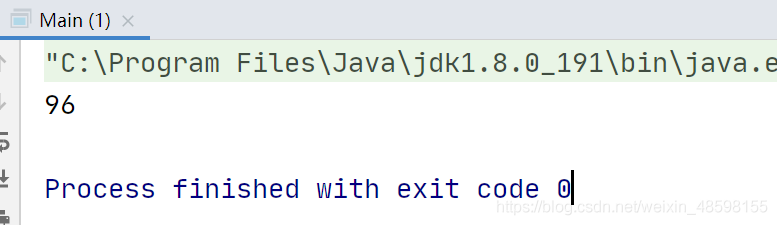# 试题 B: 寻找 2020

## 【问题描述】

• 同一行里面连续四个字符从左到右构成 2020。
• 同一列里面连续四个字符从上到下构成 2020。
• 在一条从左上到右下的斜线上连续四个字符，从左上到右下构成 2020。

``````220000
000000
002202
000000
000022
002020
``````

【答案提交】

## 【题解】

``````import java.io.BufferedReader;

public class Main {
public static void main(String[] args) throws Exception {
//缓冲流读入文件,后面路径看你放在哪个位置了
String s = null;
char[][] a = new char[];
int in = 0;
while ((s = br.readLine()) != null) {
a[in] = s.toCharArray();
in ++;
}
//定义方向，右，下，右下
int[][] dir = {{0, 1}, {1, 0}, {1, 1}};
//cut存储2020个数
int cut = 0;
for (int i = 0; i < a.length; i++) {
for (int j = 0; j < a[i].length; j++) {
if (a[i][j] != '2') continue;
for (int k = 0; k < dir.length; k++) {
//向三个方向延伸
int temp = 2;
int x = i, y = j;
for (int l = 0; l < 3; l++) {
x += dir[k];
y += dir[k];
//处理一下边界
if (x >= a.length || y >= a[x].length) break;
//拼接数字
temp = temp * 10 + a[x][y] - '0';
}
//计算出每个方向的值比对
if (temp == 2020) {
cut ++;
}
}
}
}
System.out.println(cut);
}
}

``````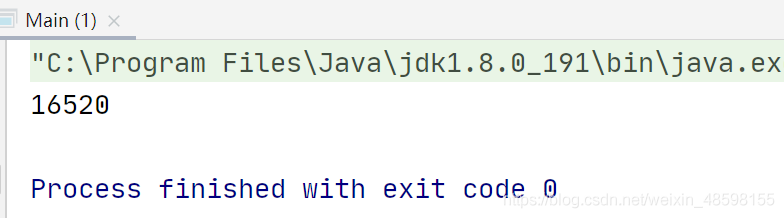# 试题 C: 跑步锻炼

【答案提交】

## 【题解】

``````public class Main {
public static void main(String[] args) {
int[] week = {1,2,3,4,5,6,7};
int weeki = 5;
int[] date = {2000,0,0};    //为了方便处理，月份和日都从0开始
int sum = 0;        //存储这20年跑步的总和
while (true) {
int year = date;
int month = date;
int day = date;
//打印日期，测试日期是否进入正轨
System.out.println(year+"年"+(month+1)+"月"+(day+1)+"日");
int s = 1;
if (week[weeki] == 1 || day == 0) {
s = 2;
}
sum += s;
if (year == 2020 && month == 9 && day == 0) {
break;
}
weeki = (weeki + 1) % 7;
//对于月份和日份构成循环
date = (day + 1) % getDayOfMonth(year,month);
date = (month + (day + 1) / getDayOfMonth(year,month)) % 12;
//月份由12月变成1月时年份+1
if (month == 11 && date == 0) date ++;
}
System.out.println(sum);
}
private static int getDayOfMonth(int year,int month) {
switch (month) {
case 0:
case 2:
case 4:
case 6:
case 7:
case 9:
case 11:
return 31;
case 3:
case 5:
case 8:
case 10:
return 30;
case 1:
if (year % 4 == 0 && year % 100 != 0 || year % 400 == 0) {
return 29;
}
return 28;
}
return 0;
}
}

``````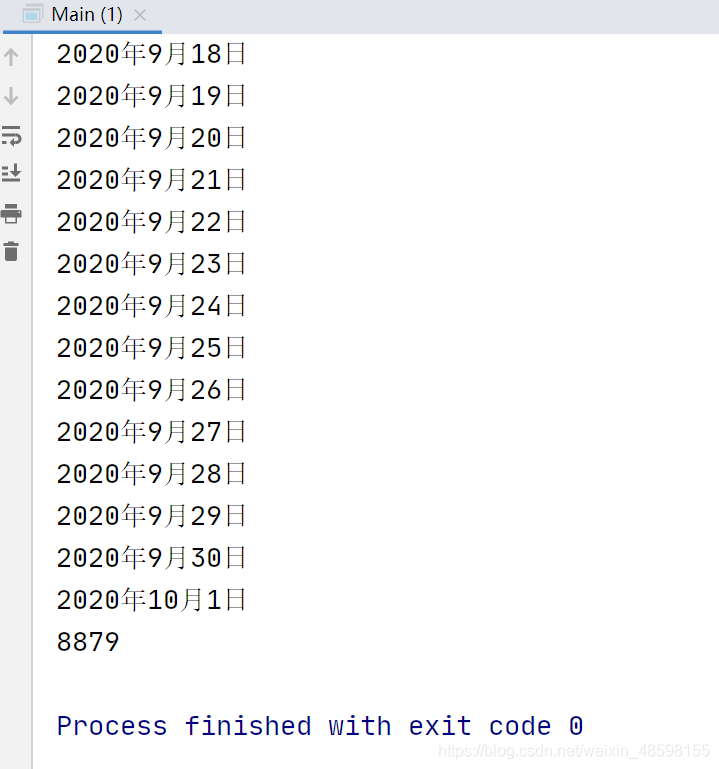# 试题 D: 平面分割

## 【问题描述】

20 个圆和 20 条直线最多能把平面分成多少个部分？
【答案提交】

## 【题解】

``````package com.cxyax.shengsai;

public class Main4 {

public static void main(String[] args) {
int n = 2;
for(int i = 1; i < 20;i++) {
n += 2 * i;
}
//      System.out.println(n);

for(int i = 1;i <= 20;i++) {
n+=39; //与圆有几个焦点，就有多焦点-1个区域
n+=i; //直线在圆外面增肌按区域数量
}
System.out.println(n);
}

}
``````

# 试题 E: 七段码

## 【问题描述】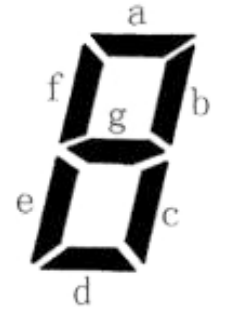• 例如：b 发光，其他二极管不发光可以用来表达一种字符。
• 例如：c 发光，其他二极管不发光可以用来表达一种字符。这种方案与上

• 例如：a, b, c, d, e 发光，f, g 不发光可以用来表达一种字符。
• 例如：b, f 发光，其他二极管不发光则不能用来表达一种字符，因为发光的二极管没有连成一片。

【答案提交】

## 【题解】``````import java.util.ArrayList;
import java.util.HashSet;

public class Main {
//res存储灯管显示方式
static HashSet<HashSet<Character>> res = new HashSet<>();
public static void main(String[] args) {
//存储所有灯管的连接关系
ArrayList<G> list = new ArrayList<>();
dfs(list,new ArrayList<G>(),0);
//结果加上7，是因为有七种方式只有一个灯管，不与其他灯管相连
System.out.println(res.size() + 7);
}
/*
深搜所有灯管连接的子集
*/
private static void dfs(ArrayList<G> list, ArrayList<G> gs, int i) {
if (i == list.size()) {
HashSet<Character> ds = new HashSet<>();
//ds获得gs里面所有的灯管
for (int j = 0; j < gs.size(); j++) {
}
//当灯管的数量比连接的数量多1，表示所有的灯管连在一起
if (ds.size() - 1 == gs.size()) {
}
return;
}
dfs(list, gs, i + 1);
dfs(list, gs, i + 1);
gs.remove(list.get(i));
}
}
/*

*/
class G {
char start;
char end;
public G (char start, char end) {
this.start = start;
this.end = end;
}
}
``````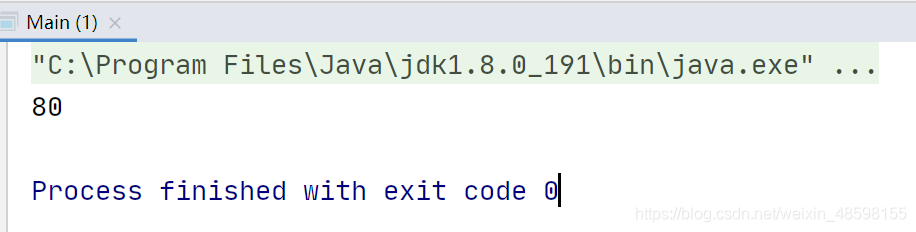# 试题 F: 成绩统计

## 【问题描述】

【输入格式】

【输出格式】

【样例输入】

``````7
80
92
56
74
88
100
0
``````

【样例输出】

``````71%
43%
``````

-- 展开阅读全文 --

« 上一篇

### 发表评论HI ! 请登录